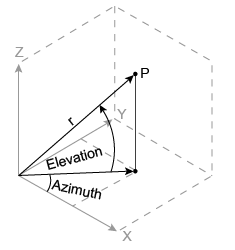sph2cart

Transform spherical coordinates to Cartesian

Description

example

[x,y,z] = sph2cart(azimuth,elevation,r) transforms corresponding elements of the spherical coordinate arrays azimuth, elevation, and r to Cartesian, or xyz, coordinates.

Examples

collapse all

Convert the spherical coordinates defined by corresponding entries in the matrices az, el, and r to Cartesian coordinates x, y, and z. These points correspond to the eight vertices of a cube.

az = [0.7854 0.7854 -0.7854 -0.7854; 2.3562 2.3562 -2.3562 -2.3562]
az = 2×4

0.7854    0.7854   -0.7854   -0.7854
2.3562    2.3562   -2.3562   -2.3562

el = [0.6155 -0.6155 0.6155 -0.6155; 0.6155 -0.6155 0.6155 -0.6155]
el = 2×4

0.6155   -0.6155    0.6155   -0.6155
0.6155   -0.6155    0.6155   -0.6155

r = 1.7321*ones(2,4)
r = 2×4

1.7321    1.7321    1.7321    1.7321
1.7321    1.7321    1.7321    1.7321

[x,y,z] = sph2cart(az,el,r)
x = 2×4

1.0000    1.0000    1.0000    1.0000
-1.0000   -1.0000   -1.0000   -1.0000

y = 2×4

1.0000    1.0000   -1.0000   -1.0000
1.0000    1.0000   -1.0000   -1.0000

z = 2×4

1.0001   -1.0001    1.0001   -1.0001
1.0001   -1.0001    1.0001   -1.0001

Input Arguments

collapse all

Azimuth angle, specified as a scalar, vector, matrix, or multidimensional array. azimuth, elevation, and r must be the same size, or any of them can be scalar.

azimuth is the counterclockwise angle in the x-y plane measured in radians from the positive x-axis.

Data Types: single | double
Complex Number Support: Yes

Elevation angle, specified as a scalar, vector, matrix, or multidimensional array. azimuth, elevation, and r must be the same size, or any of them can be scalar.

elevation is the elevation angle in radians from the x-y plane.

Data Types: single | double
Complex Number Support: Yes

Radius, specified as a scalar, vector, matrix, or multidimensional array. azimuth, elevation, and r must be the same size, or any of them can be scalar.

The length units of r can be arbitrary, and the output arrays x, y, and z use the same units.

Data Types: single | double
Complex Number Support: Yes

Output Arguments

collapse all

Cartesian coordinates, returned as arrays.

Algorithms

The mapping from spherical coordinates to three-dimensional Cartesian coordinates is

x = r .* cos(elevation) .* cos(azimuth)
y = r .* cos(elevation) .* sin(azimuth)
z = r .* sin(elevation)